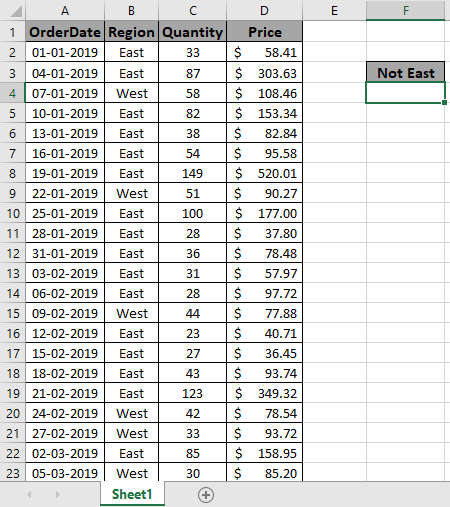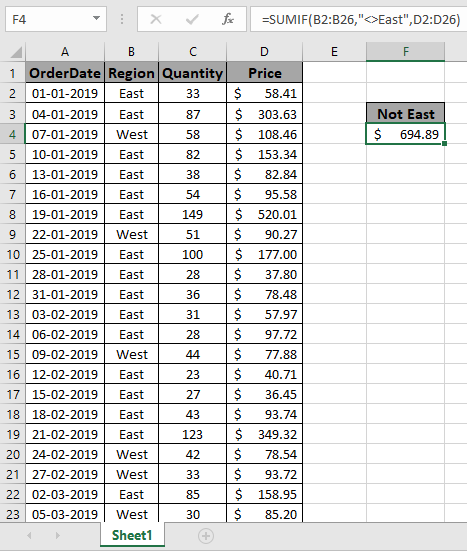# How to Sum if cell is not equal to value in Excel

In this article, we will learn about how to get the SUM value if cell value not equal to a specific value in Excel.

In simple words, when working with long data sheets, sometimes we need to extract the sum of values except where cell is not equal to the value like getting the sum of monthly expense where just a specific day’s amount not been included.

Let’s get the Generic Formula for this

=SUMIF (range, “<>value”,sum_range))

range : condition on the array
value : value to avoid
sum_range : range where sum is needed.

Let’s understand this function using it in an example.

Here we have Order from different regions with there respective quantity and Price.We need to get the total Price for all regions except East.

Use the formula:

=SUMIF(B2:B26,"<>East",D2:D26)

Explanation:
B2:B26 : range where formula matches values.
"<>East" : the formula now accepts all values except East.
<> : not equal symbol
A2:A26 : sum_range where amount adds up.
The formula drops down to
=SUMIF(
{"East";"East";"West";"East";"East";"East";"East";"West";"East";"East";"East";"East";"East";"West";"East";"East";"East";"East";"West";"West";"East";"West";"East";"East";"West"},
"<>East",
{58.41;303.63;108.46;153.34;82.84;95.58;520.01;90.27;177;37.8;78.48;57.97;97.72;77.88;40.71;36.45;93.74;349.32;78.54;93.72;158.95;85.2;107.97;139.6;160.82})

This formula adds up all the values corresponding to all the values except “East.As you can see this formula returns the sum if cell is not equal to a value

Hope you understood how to get the SUM if cell is not equal to value in Excel. Explore more articles on Excel SUMIF function here. Please feel free to state your query or feedback for the above article.

Related Articles:

Terms and Conditions of use

The applications/code on this site are distributed as is and without warranties or liability. In no event shall the owner of the copyrights, or the authors of the applications/code be liable for any loss of profit, any problems or any damage resulting from the use or evaluation of the applications/code.